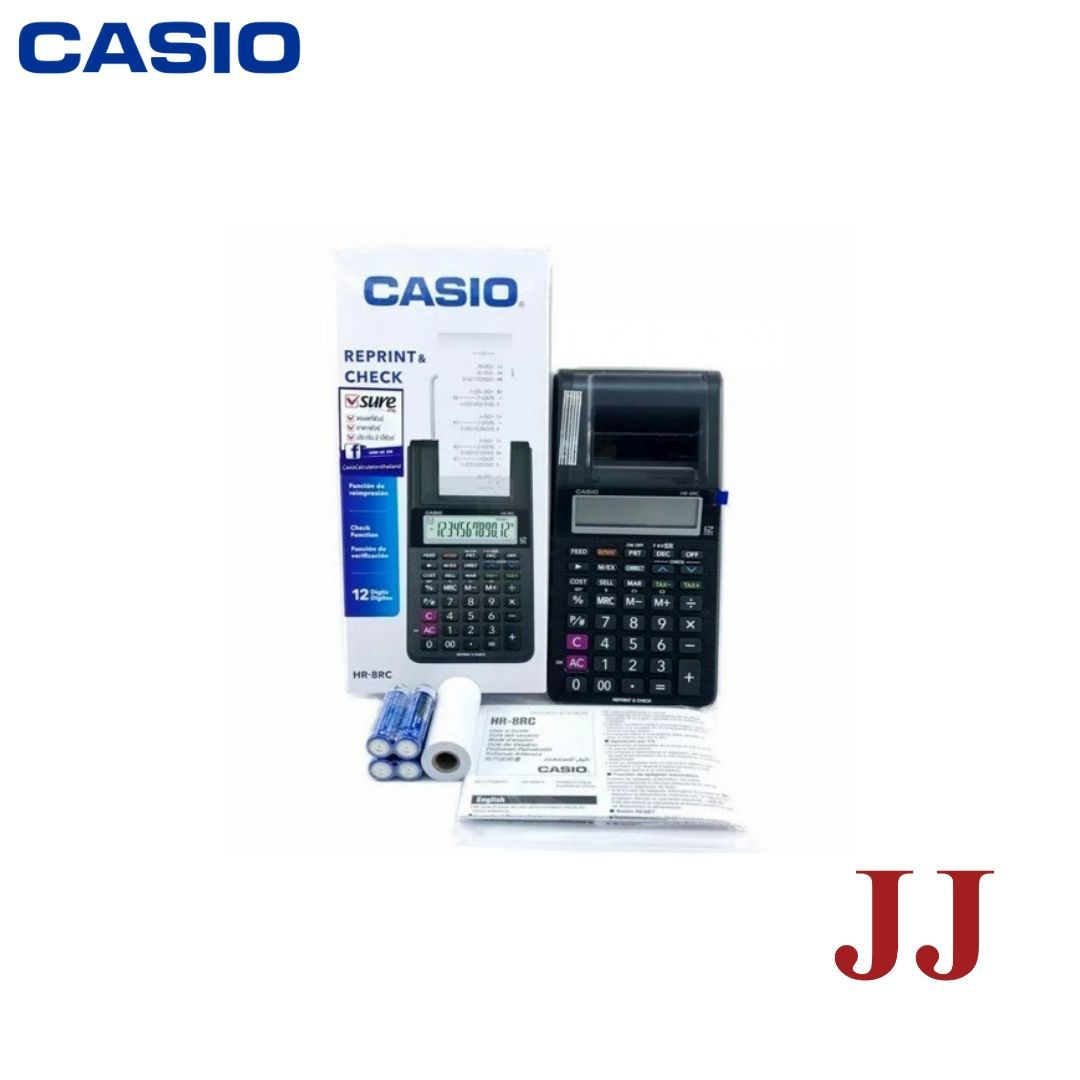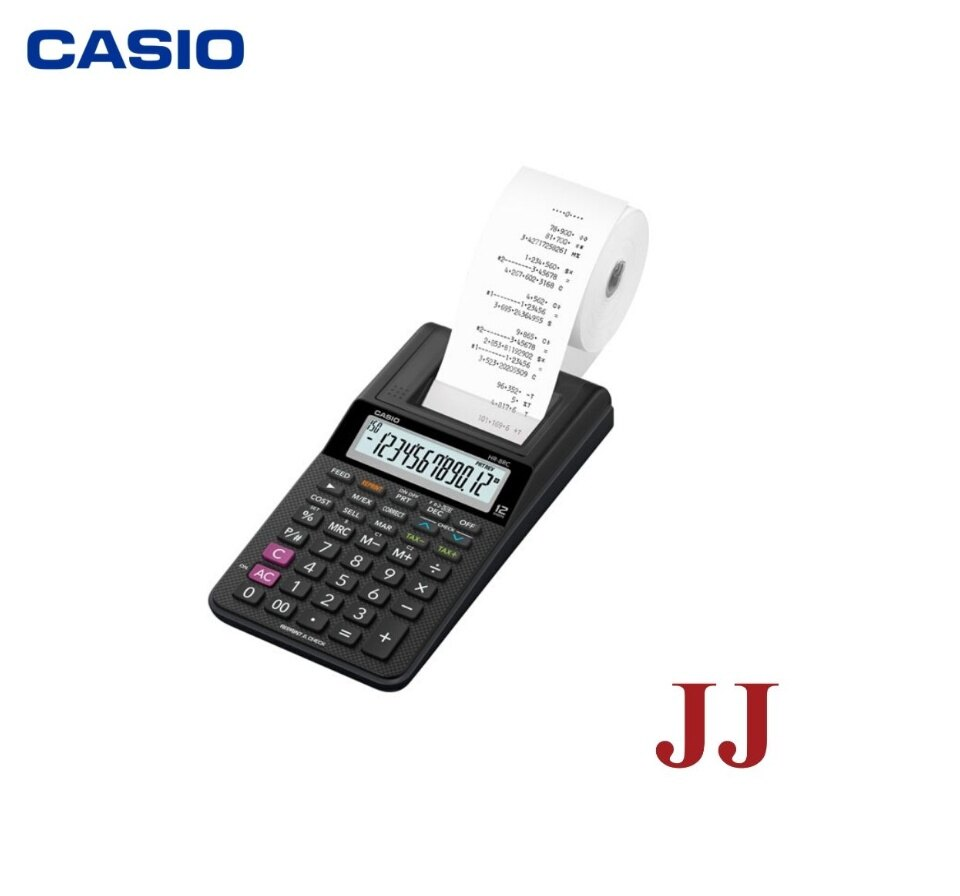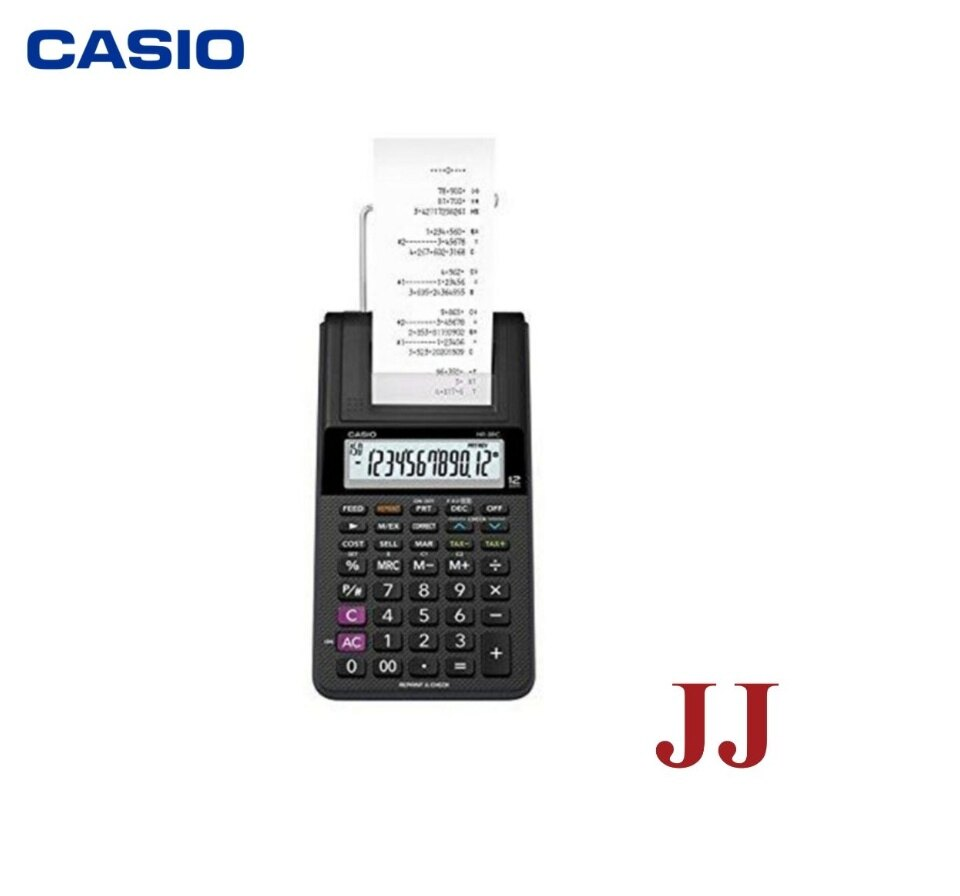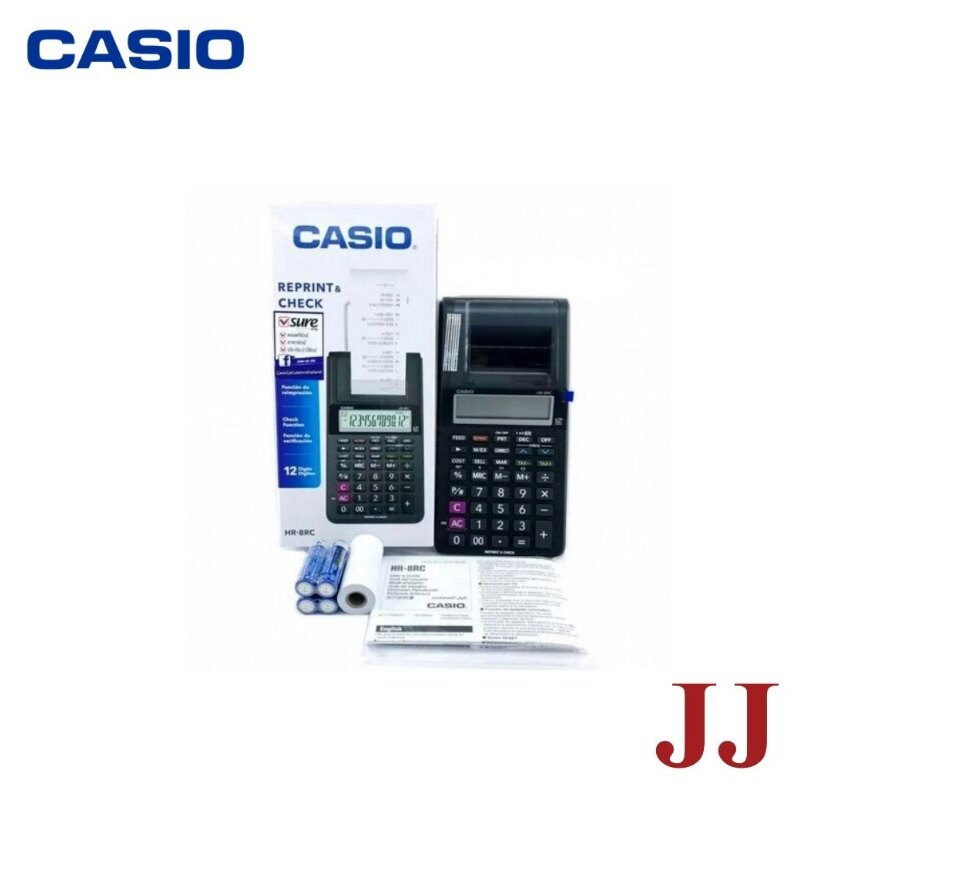# Casio HR-8RC Compact Mini 12 Digits Printing Calculator

RM 155.00
• Mini printer
• Print multiple copies of the calculation history / Print the calculation history later even in print off mode
• Displays up to 150 previous calculation steps
• Automatic calculation of price plus tax, price less tax, discount, selling price, tax amount, discount amount, and margin amount
• Currency conversion
• Calculate Cost/Sell/ Margin > Calculate the cost, selling price, or margin of profit on an item, given the other two values